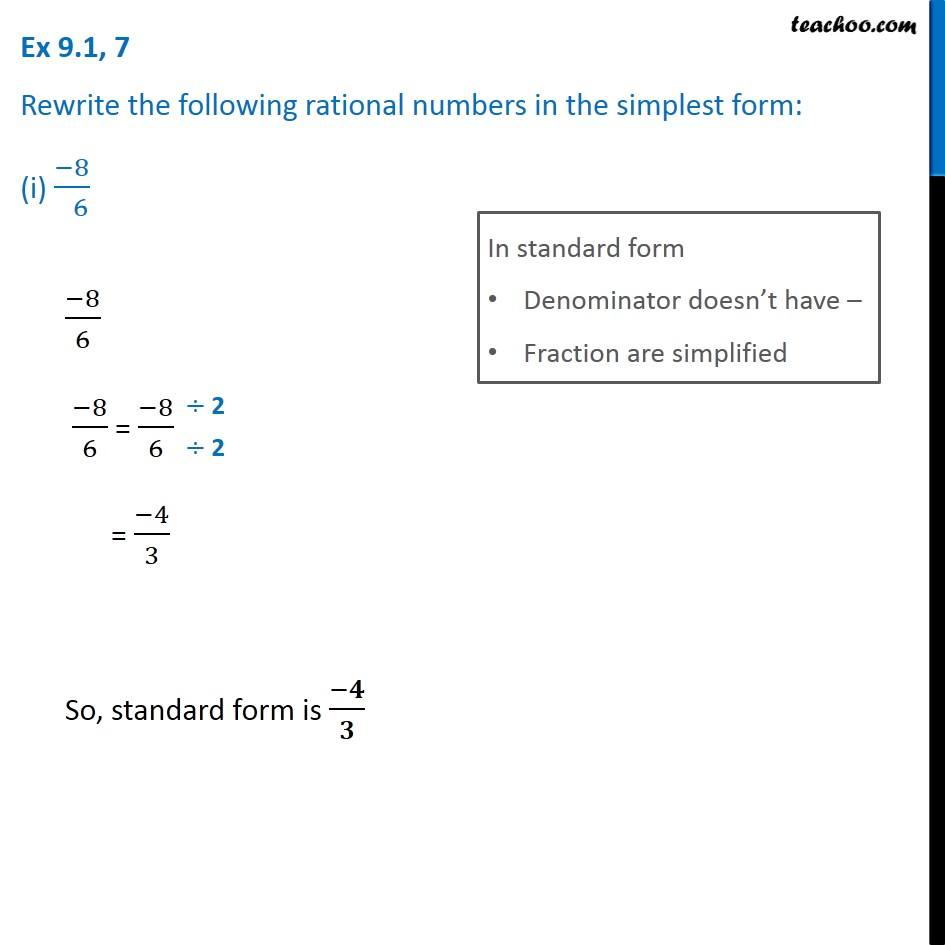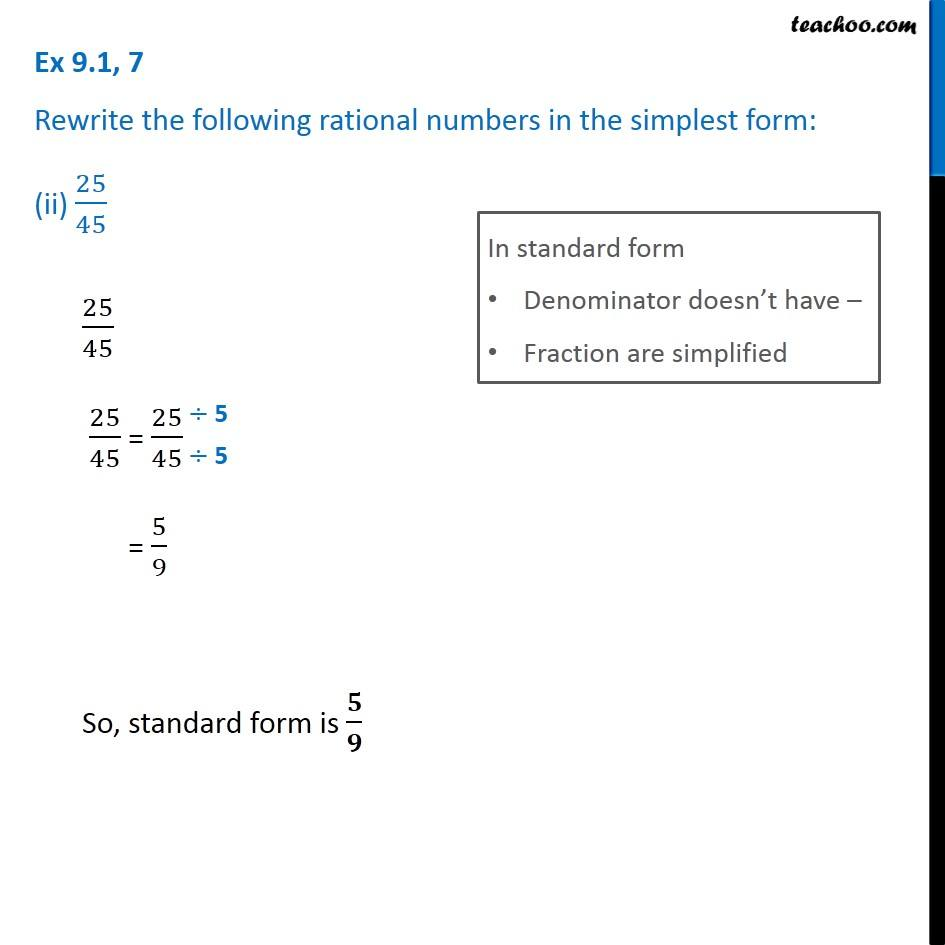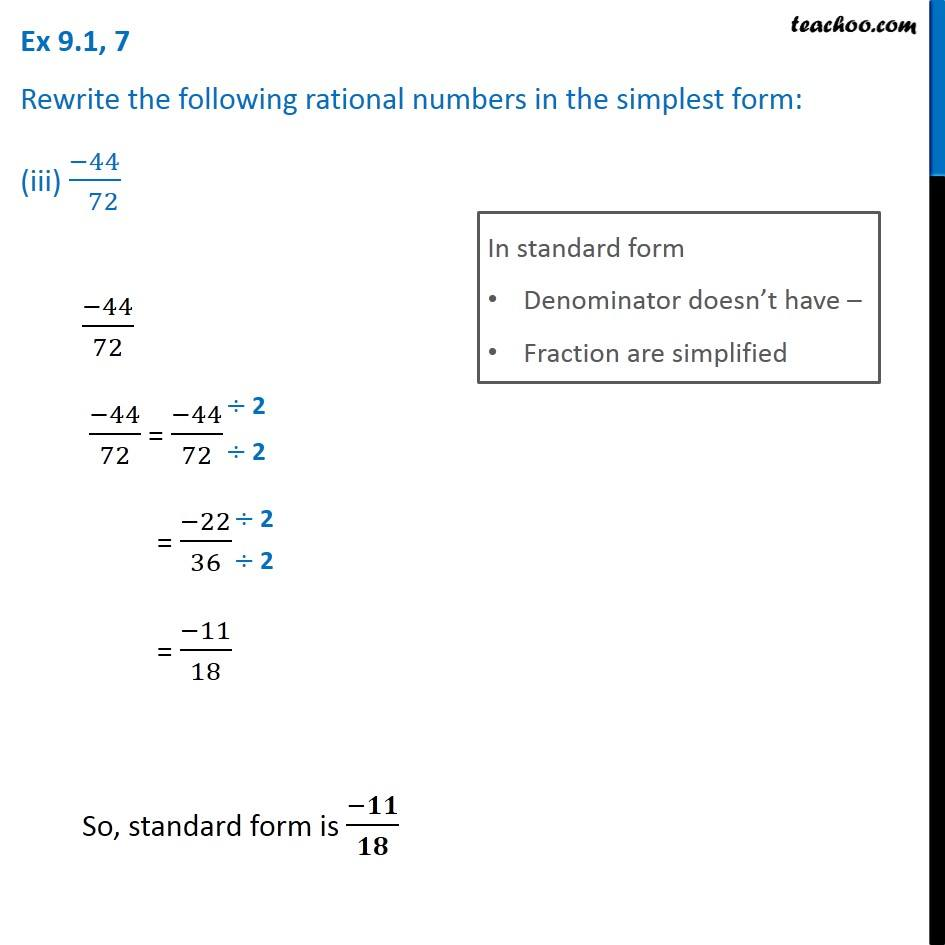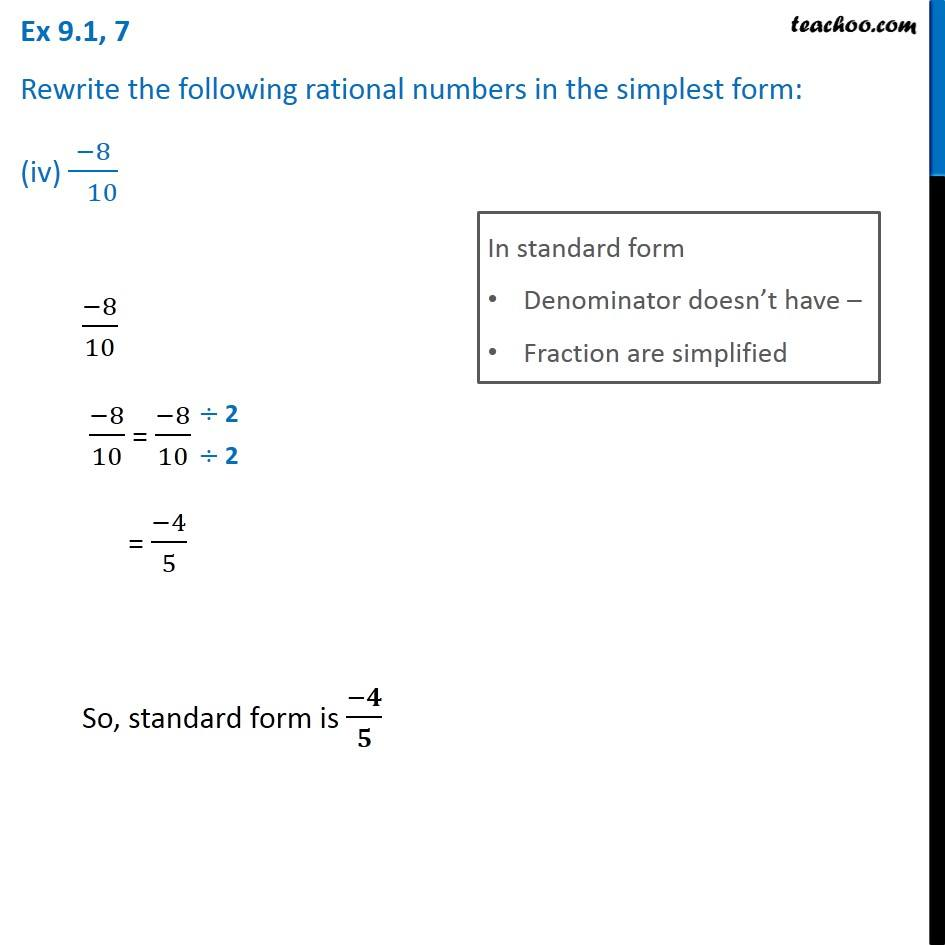Ex 9.1

Chapter 9 Class 7 Rational Numbers
Serial order wiseGet live Maths 1-on-1 Classs - Class 6 to 12

### Transcript

Ex 9.1, 7 Rewrite the following rational numbers in the simplest form: (i) (−8)/( 6) In standard form Denominator doesn’t have – Fraction are simplified (−8)/6 (−8)/6 = (−8)/6 = (−4)/3 So, standard form is (−𝟒)/𝟑 Ex 9.1, 7 Rewrite the following rational numbers in the simplest form: (ii) 25/45 In standard form Denominator doesn’t have – Fraction are simplified 25/45 25/45 = 25/45 = 5/9 So, standard form is 𝟓/𝟗 Ex 9.1, 7 Rewrite the following rational numbers in the simplest form: (iii) (−44)/( 72) In standard form Denominator doesn’t have – Fraction are simplified (−44)/72 (−44)/72 = (−44)/72 = (−22)/36 = (−11)/18 So, standard form is (−𝟏𝟏)/𝟏𝟖 Ex 9.1, 7 Rewrite the following rational numbers in the simplest form: (iv) (−8)/( 10) In standard form Denominator doesn’t have – Fraction are simplified (−8)/10 (−8)/10 = (−8)/10 = (−4)/5 So, standard form is (−𝟒)/𝟓